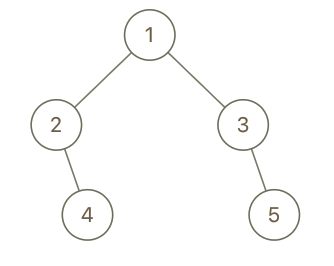## C# || How To Find Cousins In A Binary Tree Using C#The following is a module with functions which demonstrates how to find the cousins in a binary tree using C#.

1. Is Cousins – Problem Statement

Given the root of a binary tree with unique values and the values of two different nodes of the tree x and y, return true if the nodes corresponding to the values x and y in the tree are cousins, or false otherwise.

Two nodes of a binary tree are cousins if they have the same depth with different parents.

Note that in a binary tree, the root node is at the depth 0, and children of each depth k node are at the depth k + 1.

Example 1:``` Input: root = [1,2,3,4], x = 4, y = 3 Output: false ```

Example 2:``` Input: root = [1,2,3,null,4,null,5], x = 5, y = 4 Output: true ```

Example 3:``` Input: root = [1,2,3,null,4], x = 2, y = 3 Output: false ```

2. Is Cousins – Solution

The following is a solution which demonstrates how to determine if x and y values are cousins in a binary tree.

The idea here is to explore each path, finding the node values that represent x and y.

Once both values are found, save the depth and parent node, and determine if they are cousins

``` 2. Is Cousins - Solution C# // ============================================================================ // Author: Kenneth Perkins // Date: Oct 17, 2021 // Taken From: http://programmingnotes.org/ // File: Solution.cs // Description: Determines if x and y are cousins in a binary tree // ============================================================================ /** * Definition for a binary tree node. * public class TreeNode { * public int val; * public TreeNode left; * public TreeNode right; * public TreeNode(int val=0, TreeNode left=null, TreeNode right=null) { * this.val = val; * this.left = left; * this.right = right; * } * } */ public class Solution { // For the x parent and depth values private int xParent = -1; private int xDepth = -1; // For the y parent and depth values private int yParent = -1; private int yDepth = -1; public bool IsCousins(TreeNode root, int x, int y) { Search(root, x, y, root.val, 0); // Check for success condition return xParent != yParent && xDepth == yDepth; } private void Search(TreeNode node, int x, int y, int parent, int depth) { // Values have been found if (xParent != -1 && yParent != -1) { return; } if (node == null) { return; } if (node.val == x) { // Set the x parent and depth values xParent = parent; xDepth = depth; } else if (node.val == y) { // Set the y parent and depth values yParent = parent; yDepth = depth; } else { // Keep exploring along branches for the success condition Search(node.left, x, y, node.val, depth + 1); Search(node.right, x, y, node.val, depth + 1); } } }// http://programmingnotes.org/ 12345678910111213141516171819202122232425262728293031323334353637383940414243444546474849505152535455565758596061 // ============================================================================//    Author: Kenneth Perkins//    Date:   Oct 17, 2021//    Taken From: http://programmingnotes.org///    File:  Solution.cs//    Description: Determines if x and y are cousins in a binary tree// ============================================================================/** * Definition for a binary tree node. * public class TreeNode { *     public int val; *     public TreeNode left; *     public TreeNode right; *     public TreeNode(int val=0, TreeNode left=null, TreeNode right=null) { *         this.val = val; *         this.left = left; *         this.right = right; *     } * } */public class Solution {    // For the x parent and depth values    private int xParent = -1;    private int xDepth = -1;     // For the y parent and depth values    private int yParent = -1;    private int yDepth = -1;     public bool IsCousins(TreeNode root, int x, int y) {        Search(root, x, y, root.val, 0);         // Check for success condition        return xParent != yParent && xDepth == yDepth;    }     private void Search(TreeNode node, int x, int y, int parent, int depth) {        // Values have been found        if (xParent != -1 && yParent != -1) {            return;        }         if (node == null) {            return;        }         if (node.val == x) {            // Set the x parent and depth values            xParent = parent;            xDepth = depth;        } else if (node.val == y) {            // Set the y parent and depth values            yParent = parent;            yDepth = depth;        } else {            // Keep exploring along branches for the success condition            Search(node.left, x, y, node.val, depth + 1);            Search(node.right, x, y, node.val, depth + 1);        }    }}// http://programmingnotes.org/ ```

QUICK NOTES:
The highlighted lines are sections of interest to look out for.

The code is heavily commented, so no further insight is necessary. If you have any questions, feel free to leave a comment below.

Once compiled, you should get this as your output for the example cases:

``` false true false ```